# Weil algebra of a Lie algebra

(diff) ← Older revision | Latest revision (diff) | Newer revision → (diff)

Letbe a connected Lie group with Lie algebra. The Weil algebraofwas first introduced in a series of seminars by H. Cartan [a1], in part based on some unpublished work of A. Weil. As a differential graded algebra, it is given by the tensor product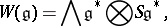where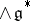and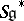denote the exterior and symmetric algebras, respectively (cf. also Exterior algebra; Symmetric algebra).

The Weil algebra and its generalizations have been studied extensively by F.W. Kamber and Ph. Tondeur [a3] [a4]. Let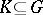be a maximal compact subgroup, withdenoting the Lie algebra of. The relative Weil algebra foris defined byWith regards to the universal classifying bundle(cf. also Bundle; Classifying space; Universal space), there are canonical isomorphisms in cohomologywhere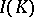denotes the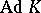-invariant polynomials. For a given integer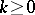, one has the ideal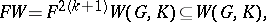generated by, for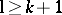. This leads to the truncated Weil algebra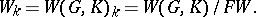The cohomology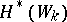plays a prominent role in the study of secondary characteristic classes (cf. also Characteristic class) of foliations and foliated bundles [a3] (see also [a2]).

How to Cite This Entry:
Weil algebra of a Lie algebra. Encyclopedia of Mathematics. URL: http://encyclopediaofmath.org/index.php?title=Weil_algebra_of_a_Lie_algebra&oldid=14594
This article was adapted from an original article by James F. Glazebrook (originator), which appeared in Encyclopedia of Mathematics - ISBN 1402006098. See original article